## Auto-Encoding Variational Bayes

In my previous post on variational inference, I gave a quick foundation of the theory behind variational auteoncoders (VAEs). The dominant lay understanding of VAEs is that they’re models which allow you to take map an image to a smaller latent space and manipulate that image in meaningful ways by changing the values of the latent space. Most machine learning tutorials are centered around this interpretation. However, I feel this short-sells their meaning (and makes people say “just use a GAN instead”, which is disappointing). It is more correct, and interesting, to say that VAEs are an implementation of variational inference which allows use of arbitrarily complex functions (read: neural networks) as approximating distributions.

Like the previous post, a lot of this information was pulled from the Stanford Probabilistic Graphical Models Course, but it’s also worth reading the original (if opaque) Kingma & Welling Paper, watching this video (starting at 7 minutes or so), and reading this StackOverflow post.

Throughout this post,refers to an input,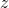refers to latent variables,refers to an actual distribution, andrefers to an approximating distribution.

In a variational autoencoder, we have a (true) equation, and want to estimate our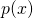.

In order to do this, the first thing we do is re-formulate the VAE objective. We still use the upper bound:Which, in our variables, is:We note that KL is always greater than or equal to zero, so we remove the intractable first KL term. We then expand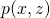with the probability chain rule (and logarithm product rule).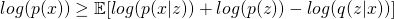Exploit the linearity of expectation: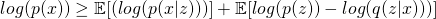Flip the signs and collect the right two terms as a KL divergence (asmarginalizes out, this is a straightforward algebraic manipulation of the KL divergence):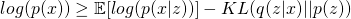Which is our objective function for the variational autoencoder. We can pause here for a minute and take a look at how this lines up with the common interpretation of our variational autoencoder. We note that there is a, which corresponds to our encoder network, a, which corresponds to our prior (a set of independent multivariate unit gaussians), and a, which corresponds to our decoder network. The expected value operator is also significant, as it means we never actually need to know the value of the probability. The expected value is simply the output of the decoder network when all latent variables are at their mean value, and making the probability ofhigher under this distribution corresponds to just making the input and output images match more closely!

There is one (not small) detail missing before we can perform gradient descent. Sinceare random variables, we can’t differentiate through them to train the encoder and decoder network. This is what the reparameterization trick is for. In the reparameterization trick, we produce two outputs in our latent space (mean and standard deviation) and treat them as deterministic. That is, these are the outputs we want to learn. But we have a third, random, variable that we multiply sigma by which is drawn from a unit normal distribution. Since this isn’t learned, we can still backpropagate through the network as desired despite having random variables within it.

## A rapid look at variational inference with KL divergence

I’ve been spending some time reading up on variational autoencoders (VAEs), which are a paradigm of machine learning that draws some interesting parallels with Bayesian Inference. In order to understand why VAEs worked (aside from just saying neural networks and refusing to think further), I had to get at least a surface level understanding of variational methods.

This information is rephrased from the Stanford Course on Probabilistic Graphical Models here.

Variational inference seeks to cast (often intractable) inference problems as optimization problems. There are some differences between how various fields refer to inference, but in this case inference signifies extracting information from the probabilistic model. This information may be the probability of a given variable after we sum everything else out (marginal inference) or the most likely assignment to the variables in the model (maximum a-posteriori).

Performing variational inference requires an approximating family of distributions,, and an optimization objective,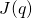. One type of variational inference, called “mean field” uses the product of many univariate distributions as the approximating family. However, there are many other options (for example, VAEs use neural networks as approximating families).

For the optimization objective,, we typically use the KL divergence, which is defined as:The KL divergence is not symmetric (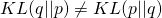) but is always greater than 0 whenand 0 when. This second point is important.

As a specific problem, we showto be a joint distribution of many variables, which (for purely pedagogical reasons) is the normalized version of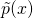.represents the parameters of the distribution, andis the normalizing distribution.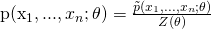From here, we note that we can’t calculateor, but we can still play with our optimization objective.We note that, so it follows that our optimization objective can be expressed as:And re-formed as:The KL divergence betweenand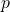is still intractable. HOWEVER, since KL divergences are always greater than or equal to zero, our KL divergence betweenand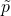(i.e.,) is tractable, and is a lower bound on the probability of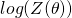. This is called the Variational Lower Bound (VLBO) or more commonly (in my reading) the Evidence Lower Bound (ELBO).

How is this equation useful? It shows up in the mathematics for the variational autoencoder, which I describe in the next post. It also shows up all sorts of other places that I haven’t covered.